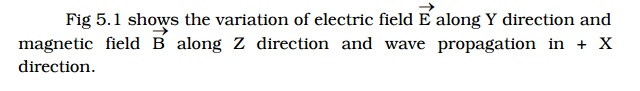Home | | Physics | Characteristics of electromagnetic waves

# Characteristics of electromagnetic waves

In an electromagnetic wave, electric and magnetic field vectors are at right angles to each other and both are at right angles to the direction of propagation.

## Electromagnetic waves

According to Maxwell, an accelerated charge is a source of electromagnetic radiation.In an electromagnetic wave, electric and magnetic field vectors are at right angles to each other and both are at right angles to the direction of propagation. They possess the wave character and propagate through free space without any material medium. These waves are transverse in nature.2. Characteristics of electromagnetic waves

(i) Electromagnetic waves are produced by accelerated charges.

(ii) They do not require any material medium for propagation.

(iii) In an electromagnetic wave, the electric Vector(E) and magnetic Vector(B) field vectors are at right angles to each other and to the direction of propagation. Hence electromagnetic waves are transverse in nature.

(iv) Variation of maxima and minima in both Vector(E) and Vector(B) occur simultaneously.

(v) They travel in vacuum or free space with a velocity 3 × 108 m s−1 given by the relation C = 1/Root(µoεo)

o – permeability of free space and εo - permittivity of free space)

(vi) The energy in an electromagnetic wave is equally divided between electric and magnetic field vectors.

(vii) The electromagnetic waves being chargeless, are not deflected by electric and magnetic fields.

Study Material, Lecturing Notes, Assignment, Reference, Wiki description explanation, brief detail
Physics : Electromagnetic Waves and Wave optics : Higher Secondary(12 Std) : Characteristics of electromagnetic waves |